# vs.eyeandcontacts.com

## Chapter 11 - Force and Pressure

Multiple Choice Questions
Question 1: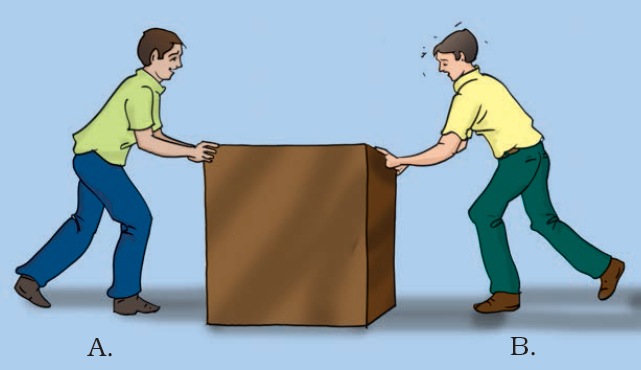In Fig 11.1, two boys A and B are shown applying force on a block. If the block moves towards the right, which one of the following statements is correct?
a) Magnitude of force applied by A is greater than that of B.
b) Magnitude of force applied by A is smaller than that of B.
c) Net force on the block is towards A.
d) Magnitude of force applied by A is equal to that of B.
a) Magnitude of force applied by A is greater than that of B.

Question 2: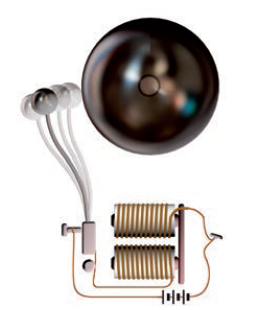In the circuit shown in Fig.11.2, when the circuit is completed, the hammer strikes the gong. Which of the following force is responsible for the movement of hammer?
a) gravitational force alone
b) electrostatic force alone
c) magnetic force alone
d) frictional force alone
c) magnetic force alone

Question 3: During dry weather, while combing hair, sometimes we experience hair flying apart. The force responsible for this is
a) force of gravity.
b) electrostatic force.
c) force of friction.
d) magnetic force.
b) electrostatic force.

Question 4: Fig.11.3 shows a container filled with water. Which of the following statements is correct about pressure of water?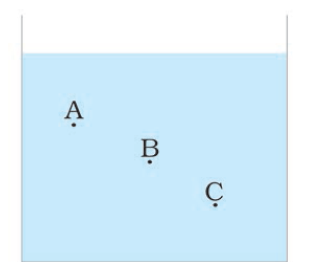a) Pressure at A> Pressure at B> Pressure at C
b) Pressure at A=Pressure at B=Pressure at C
c) Pressure at A< Pressure at B>Pressure at C
d) Pressure at A< Pressure at B<Pressure at C
d) Pressure at A< Pressure at B<Pressure at C

Question 5: Two objects repel each other. This repulsion could be due to
a) frictional force only
b) electrostatic force only
c) magnetic force only
d) either a magnetic or an electrostatic force
d) either a magnetic or an electrostatic force

Question 6: Which one of the following forces is a contact force?
a) force of gravity
b) force of friction
c) magnetic force
d) electrostatic force
b) force of friction

Question 7: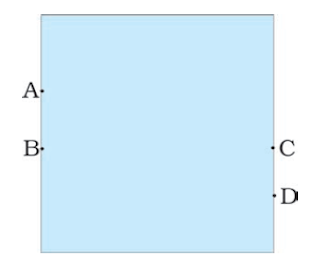A water tank has four taps fixed at points A, B, C, D as shown in Fig. 11.4. The water will flow out at the same pressure from taps at
a) B and C
b) A and B
c) C and D
d) A and C
a) B and C

Question 8: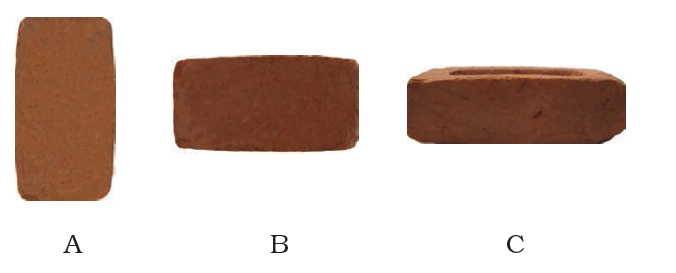A brick is kept in three different ways on a table as shown in Fig. 11.5. The pressure exerted by the brick on the table will be
a) maximum in position A
b) maximum in position B
c) maximum in position C
d) equal in all cases.
a) maximum in position A

Question 9: A ball of dough is rolled into a flat chapatti. Name the force exerted to change the shape of the dough.
The force exerted to change the shape of the dough is muscular force.

Question 10: Where do we apply a force while walking?
While we walk, we apply force on the ground.

Question 11: A girl is pushing a box towards east direction. In which direction should her friend push the box so that it moves faster in the same direction?
A girl is pushing a box towards east direction, so her friend should also push the box in east direction so that it moves faster in the same direction because the net force will increase (F1 + F2).

Question 12: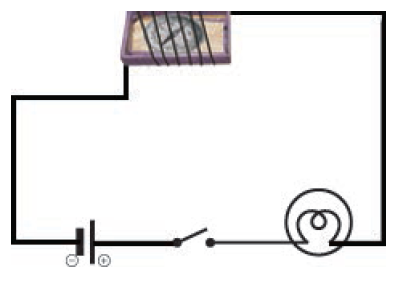In the circuit shown in Fig.11.6, when the key is closed, the compass needle placed in the match box deflects. Name the force which causes this deflection.
The compass needle placed in match box deflects, the force which causes this deflection is magnetic force. Magnetic force is the force exerted by magnets.

Question 13: During dry weather, clothes made of synthetic fibre often stick to the skin. Which type of force is responsible for this phenomenon?
During dry weather, clothes made of synthetic fibre often stick to the skin, the force responsible for this phenomenon is electrostatic.

Question 14: While sieving grains, small pieces fall down. Which force pulls them down?
While sieving grains, small piece fall down, the force pull them down is gravity (force of gravity or gravitational force). This is because gravitational force is the force which is exerted by Earth on all objects. It pulls all objects towards the Earth.

Question 15: Does force of gravity act on dust particles?
Yes, force of gravity acts on dust particles because the Earth pulls everything towards it.

Question 16: A gas filled balloon moves up. Is the upward force acting on it larger or smaller than the force of gravity?
Upward force is larger than the force of gravity.

Question 17: Does the force of gravitation exist between two astronauts in space?
Yes, the force of gravitation exists between two astronauts in space because Newton's gravitational law states that there is always an attraction force exist between any two masses in the universe.

Question 18: A chapati maker is a machine which converts balls of dough into chapati’s. What effect of force comes into play in this process?
A chapati maker is a machine which converts balls of dough into chapati’s, the effect of force that comes into play in this process is the force changes the shape of dough.

Question 19: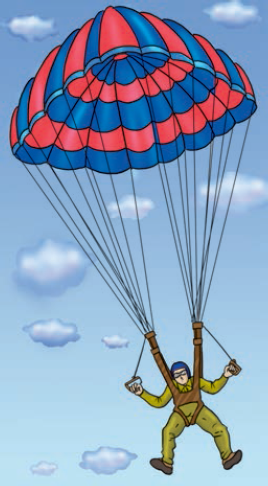Fig.11.7 shows a man with a parachute. Name the force which is responsible for his downward motion. Will he come down with the same speed without the parachute?
The force responsible for his downward motion is gravity (force of gravity or gravitational force). No, he will not come down with same speed without the parachute because the friction exerted by the man will be less. So his speed will be higher without parachute.

Question 20: Two persons are applying forces on two opposite sides of a moving cart. The cart still moves with the same speed in the same direction. What do you infer about the magnitudes and direction of the forces applied.
Both the forces are of equal magnitudes and applied in the opposite directions.

Question 21: Two thermocol balls held close to each other move away from each other. When they are released, name the force which might be responsible for this phenomenon. Explain.
The force which might be responsible for this phenomenon is electrostatic force. Both the thermocol balls are charged body so they move away due to repulsion between similar charges.

Question 22: Fruits detached from a tree fall down due to force of gravity. We know that a force arises due to interaction between two objects. Name the objects interacting in this case.
The objects interacting in this case are Earth and fruits.

Question 23: A man is pushing a cart down a slope. Suddenly the cart starts moving faster and he wants to slow it down. What should he do?
The man should apply the force in opposite direction by pulling it so as to slow it down because if he pushes it, the net force will increase but if he pulls it, the net force will decrease.

Question 24: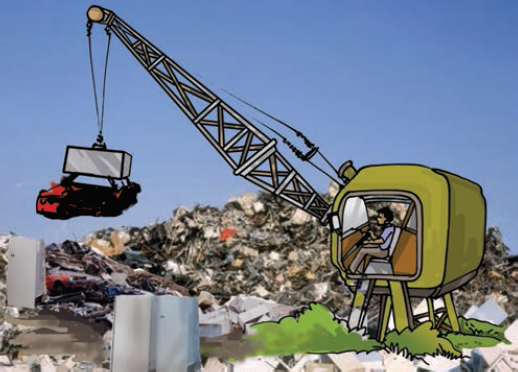Fig. 11.8 shows a car sticking to an electromagnet. Name the forces acting on the car? Which one of them is larger?
There are 2 forces acting on it. They are magnetic force and force of gravity. The magnetic and force of gravity. Magnetic force acts in the upward direction and force of gravity or the weight of the car acts in downward direction. Magnetic force is larger than the force of gravity.

Question 25: An archer shoots an arrow in the air horizontally. However, after moving some distance, the arrow falls to the ground. Name the initial force that sets the arrow in motion. Explain why the arrow ultimately falls down.
The archer stretches the string of the bow by applying muscular force. In the process the shape of the bow changes. When the string is released, it regains its original position that provides the initial force to set the arrow in motion. The force of gravity that acts on the arrow in the downward direction brings it to the ground.

Question 26: It is difficult to cut cloth using a pair of scissors with blunt blades. Explain.
Blunt blades have larger area in comparison to the sharp-edged blades. So, the applied force produces a lower pressure in case of blunt blades that makes it difficult to cut the cloth.

Question 27: Two rods of the same weight and equal length have different thickness. They are held vertically on the surface of sand as shown in Fig.11.9. Which one of them will sink move? Why?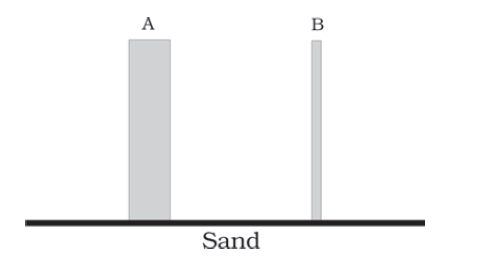Answer: Rod B will go deeper as it has a smaller area of contact, therefore the same force (weight of the rod) produces more pressure. In case of rod A the same force produces less pressure.

Question 28: Two women are of the same weight. One wears sandals with pointed heels while the other wears sandals with flat soles. Which one would feel more comfortable while walking on a sandy beach? Give reasons for your answer.
The woman wearing sandals with flat soles will feel more comfortable while walking on the sandy beach. The flat soles have larger area compared to the sandals with pointed heels. Since the two women are of the same weight, they will apply same force on the ground. Therefore, the pressure exerted by the pointed heels will be more compared to that with sandals having flat soles. As a result the pointed heel sandals will sink more in the sand than the flat sole sandals. Therefore, walking with flat sole sandals will be more comfortable.

Question 29: It is much easier to burst an inflated balloon with a needle than by a finger. Explain.
It is much easier to burst an inflated balloon with a needle than by a finger because when we prick the surface of an inflated balloon with a needle it exerts a larger pressure because it has a smaller area of contact compared to the finger. The large pressure pierces the surface of the balloon easily.

Question 30: Observe the vessels A, B, C and D shown in Fig.11.10 carefully.Volume of water taken in each vessel is as shown. Arrange them in the order of decreasing pressure at the base of each vessel. Explain.